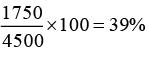Courses

# Quant Mock Test - 16

## 15 Questions MCQ Test Mock Test Series for CLAT 2020 | Quant Mock Test - 16

Description
This mock test of Quant Mock Test - 16 for CLAT helps you for every CLAT entrance exam. This contains 15 Multiple Choice Questions for CLAT Quant Mock Test - 16 (mcq) to study with solutions a complete question bank. The solved questions answers in this Quant Mock Test - 16 quiz give you a good mix of easy questions and tough questions. CLAT students definitely take this Quant Mock Test - 16 exercise for a better result in the exam. You can find other Quant Mock Test - 16 extra questions, long questions & short questions for CLAT on EduRev as well by searching above.
QUESTION: 1

### Directions: Study the following pie chart carefully and answer the questions given below: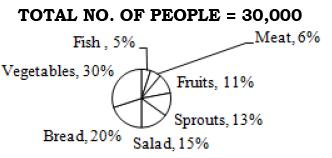Q. What is the total number of people preferring vegetables and those preferring sprouts?

Solution:

No. of people prefer vegetables = 30000 x 30% = 9000.
No. of people preferring sprouts = 30000 x 13% = 3900.
Required total no. = 9000 + 3900 = 12900

QUESTION: 2

### Directions: Study the following pie chart carefully and answer the questions given below:Q. What is the difference between the total number of people preferring meat to the total number of people preferring fish?

Solution:

Difference between the total no. of people preferring meat to the total no. of people preferring fish = 30000 x (6% - 5%) = 300.

QUESTION: 3

### Directions: Study the following pie chart carefully and answer the questions given below:Q. People preferring fruits are approximately what percent of the people preferring vegetables?

Solution:

No. of people preferring fruits = 30000 x 11% = 3300.
No. of people preferring vegetables = 9000. Required %=3300/9000 x 100 = 36.66 = 37 (approx.)

QUESTION: 4

Directions: Study the following pie chart carefully and answer the questions given below:Q. Out of the total sample population, how many people have given preference for fish?

Solution:

No. of people preferring fish = 30000 x 5% = 1500

QUESTION: 5

Directions: Study the following pie chart carefully and answer the questions given below:Q. What is the ratio of the number of people preferring meat to the number of people preferring salad?

Solution:

No. of people preferring meat = 30000 x 6% = 1800.
No. of people preferring salad =30000 x 15% = 4500
Required ratio = 1800 : 4500 = 2 : 5

QUESTION: 6

Directions: Study the following table carefully to answer the questions that follow:
Over the years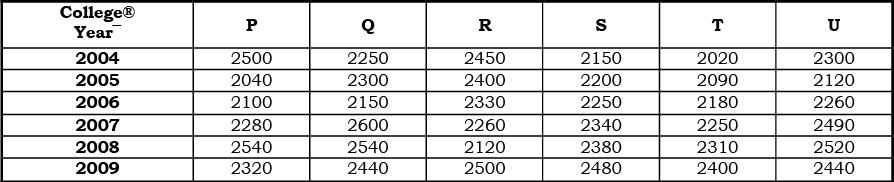Q. What is the percent increase in the number of students in college T in the year 2007 from the previous year? (Rounded off to two digit after decimal)

Solution: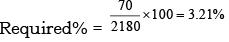QUESTION: 7

Directions: Study the following table carefully to answer the questions that follow:
Over the yearsQ. What is the total number of students from all the colleges together in the year 2005?

Solution:
QUESTION: 8

Directions: Study the following table carefully to answer the questions that follow:
Over the yearsQ. What is the average number of students in all the colleges together in the year 2004? (Rounded off to the nearest integer)

Solution:

Avg =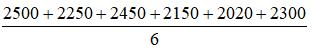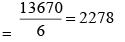QUESTION: 9

Directions: Study the following table carefully to answer the questions that follow:
Over the yearsQ. The number of students in college P in the year 2008 forms approximately what percent of the total number of students in that college from all the years together?

Solution: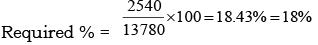QUESTION: 10

Directions: Study the following table carefully to answer the questions that follow:
Over the yearsQ. What is the ratio of the number of students in college S in the year 2006 and 2009 together to the total number of students in that college U from the same years?

Solution:

Required ratio = (2250 + 2480) : (2260 + 2440)
= 4730 : 4700 = 473 : 470

QUESTION: 11

Directions: Study the following pie-chart carefully to answer the questions that follow.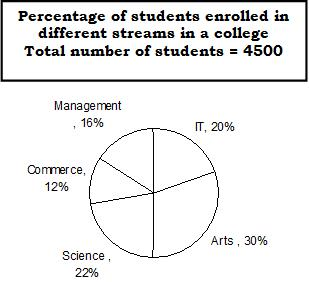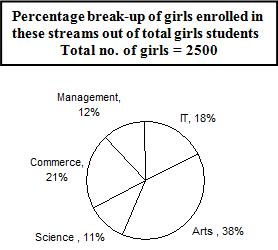Q. What is the total number of boys enrolled in management and IT together?

Solution:

Total number of girls enrolled in Management and IT together = 30% 2500 = 750
Total number of students enrolled in Management and IT together = 36% of 4500 = 1620.
Required number of boys = 1620 - 750 = 870

QUESTION: 12

Directions: Study the following pie-chart carefully to answer the questions that follow.Q. What is the ratio of the number of girls enrolled in Arts to the number of boys enrolled in Science?

Solution:

Number of girls enrolled in Arts = 38% of 2500 = 950.
Number of girls enrolled in Science = 11% of 2500 = 275.
Number of boys enrolled in Science = 22% of 4500 – 275 = 990 – 275 = 715
Required ratio = 950 : 715 = 190 : 143

QUESTION: 13

Directions: Study the following pie-chart carefully to answer the questions that follow.Q. What is the total number of girls enrolled in Science and Commerce together?

Solution:

Required number of girls = 32% of 2500 = 800

QUESTION: 14

Directions: Study the following pie-chart carefully to answer the questions that follow.Q. If 20% of the girls enrolled in Science change their stream to Management. Then how many students will be there in management altogether?

Solution:

Number of girls enrolled in Science = 20% of 275 = 1/5 x 275 = 55
Number of students enrolled in Management = 16% of 4500 = 720
Total number of students in Management = 720 + 55 = 775

QUESTION: 15

Directions: Study the following pie-chart carefully to answer the questions that follow.Q. The number of girls enrolled in Arts, Science and Commerce forms approximately what per cent of total number of students in the college?

Solution:

The number of girls enrolled in Arts, Science and Commerce = 70% of 2500 = 1750
Required percentage =